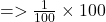## 1. Calculate the mass percent of salt in salt water if 1 gram of salt is dissolved into 99 grams of water. Show the steps in your calculatio

Question

1. Calculate the mass percent of salt in salt water if 1 gram of salt is dissolved into 99 grams of water. Show the steps in your calculation.

in progress 0
5 months 2021-08-13T09:01:19+00:00 1 Answers 18 views 0

## Answers ( )

1. Question :—

1. Calculate the mass percent of salt in salt water if 1 gram of salt is dissolved into 99 grams of water. Show the steps in your calculation.

gconcentration of solution= mass of solution (M mass of solute) ×100